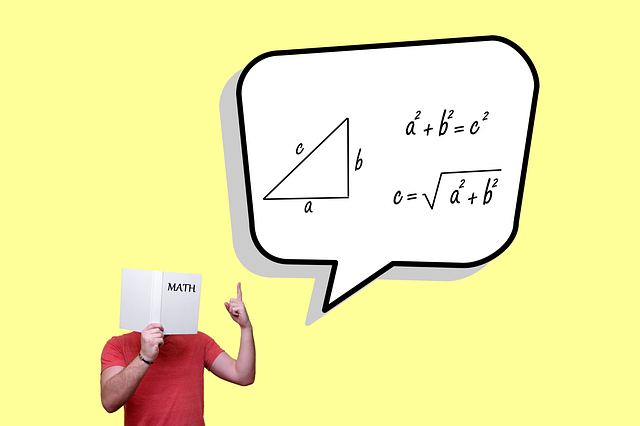Diksha Jani is a blogger likes to read novels and technical content. She also likes to explore new places

The Bayes theorem is considered the best possible way of describing the probability of any event related to any condition. This is also consider to be the case and condition of conditional probability. This particular theorem is also refer to as the formula for the calculation of the probability of causes.

In this particular system, the individuals need to calculate the probability of taking something depending upon the situation. The probability of the occurrence of an event will be calculate with the help of different kinds of conditions.

## Following is the Compressive Formula of the Bayes Theorem or Conditional Probability:

The probability of A/B is equal to the probability of B/A into the probability of A upon the probability of B.

Suppose there will be a comprehensive set of events associate with the sample space S. In that case, all these kinds of events will have a different set of probability. It is calculate using this particular type of formula. So also, the Bayes theorem comes with comprehensive proof that the students need to be very clear about indulging in the implementation of the formula very easily.

Following are some of the very basic terminologies which the students need to be clear about before implementing this particular formula:

• The hypothesis: All the events like event one, event two, event N. And several other kinds of things will be refer to as the hypothesis.
• Priori probability: This will be the probability of the hypothesis of event I.
• Posteriori probability: This will be the probability of event I/A.

## Compressive Formula

The formula mentioned above has been perfectly utilize to calculate the probability of a particular condition. Where event A will be occurring, and B event has already occurred. The derivation of this particular theorem is another very important thing to consider by the people for the random variables separately during the definition of conditional probability and density.

Being clear about all these kinds of terms is very important for the people to achieve overall goals efficiently. They can calculate things very easily. This rule can be utilize in the condition when answering the probability queries, are condition on one place of the area with one piece of evidence.

The theorem will always help govern the likelihood that one event will be based on the occurrence of some of the other events which is known as conditional probability.

It is important for the kids into this particular area to indulge in proper solving of different kinds of questions. It is associate with this rule and implementation of the things. Practice is the only thing that will help kids to become successful in solving the questions and examinations.

### In Conclusion:

This concept is also Bayes on different kinds of real-life examples. For example, utilization of the 2 dice, coins, and various other kinds of events in normal human life. Apart from this being clear about the derivation of the formula is also very important.

When they are clear about the derivation, the students will have a clear-cut idea about every element of the formula that will further allow them to make the formula right kind of decisions throughout the process.

Also, in the world of probability, kids need to be crystal clear. About the whole scenario and solve the questions without any hassle. Depending on experts from the house of Cuemath is a wonderful idea to become masters of the subject and have a good command of the probability and Bayes theorem.